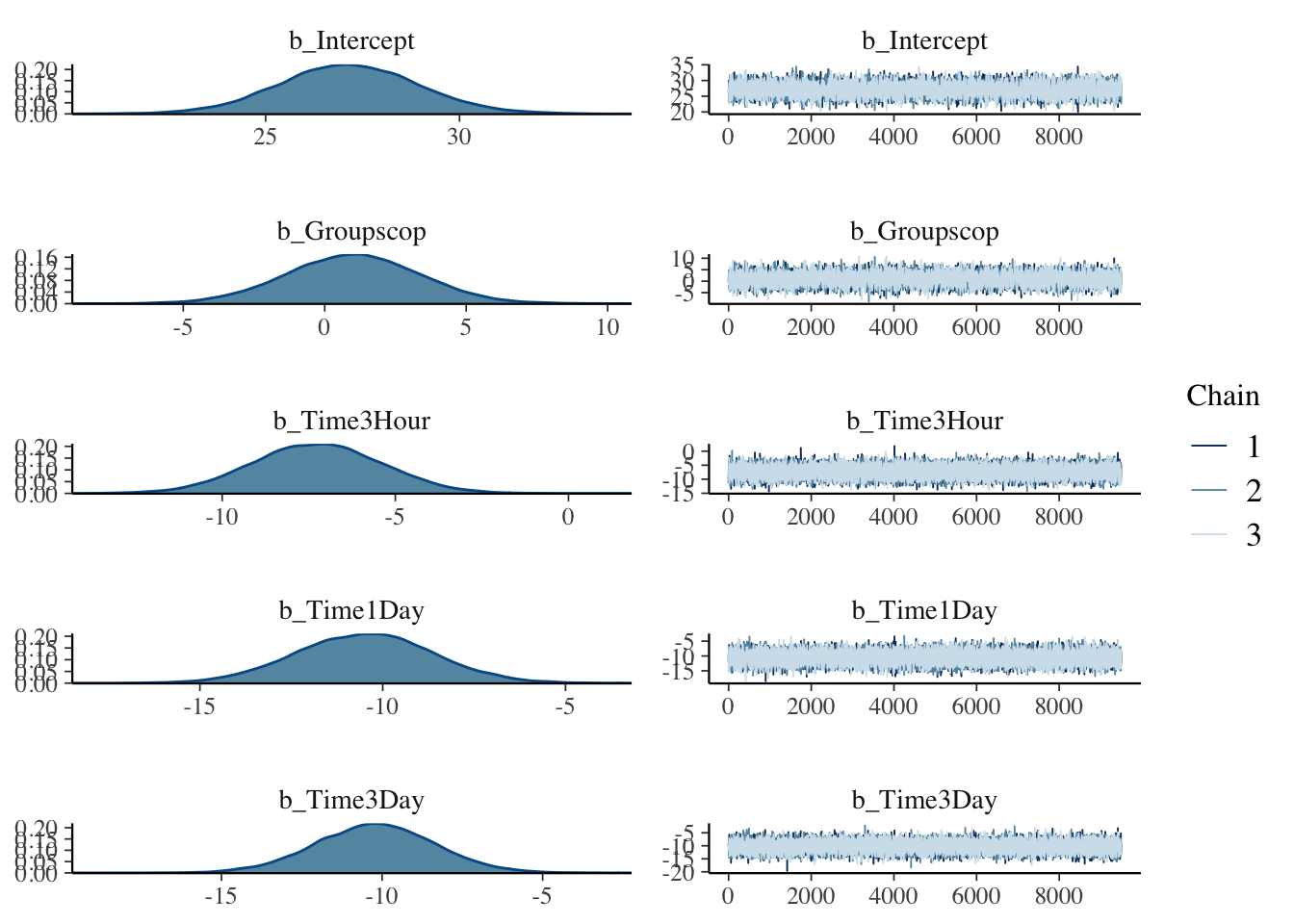We specify a Bayesian mixed effects model equivalent to that in lme4 as:

\begin{align*} y & \sim \operatorname{Normal}(\mu, \sigma_{t}) \\ \mu & = \beta_{0} + s_j + (\overrightarrow{\beta_{Time}} \times \overrightarrow{X_{Time}}) + (\overrightarrow{\beta_{Group}} \times \overrightarrow{X_{Group}}) + (\overrightarrow{\beta_{Int}} \times \overrightarrow{X_{Int}})\\ \overrightarrow{\beta_{Age}},\overrightarrow{\beta_{Time}},\overrightarrow{\beta_{Int}} & \sim \operatorname{Normal}(0, 10) \\ \beta_{0} & \sim \operatorname{Normal}(30, 5) \\ s_{j} & \sim \operatorname{Normal}(0, \sigma_{s}) \\ \sigma_{s},\sigma_{t} & \sim \operatorname{Half Student}(3,0,mad(y)) \\ \end{align*}

Overarrows are used to indicate vectors with dummy coding of categorical fixed effect variables and $$s_j$$ corresponds to the random efffects of participant j. Relatively non-committal priors are used. For the overall intercept a normal prior with mean of 30 with sd of 5 gives 95% coverage of the range 20-40. 20 is the minimum entry criteria for the study while a patient with severe MADRS score of > 40 would typically be excluded. For fixed effects, normal priors are used with an sd of 10 which allows for a range of effect sizes of size comparable to that seen in prior studies of scopolamine (e.g. Drevets and Furey (2010)) and a relatively similar local population for which we tested ketamine as an antidepressant (Sumner et al. (2020)). sd priors are specified as default half student t priors as suggested by Gelman (2006) using the median absolute deviation (mad) of y as the scale factor (Bürkner (2017)). For MCMC modelling Stan is acccessed through the brms interface (Bürkner (2017)). For computing Bayes Factors, a ROPE of range |sd/10| is used (Kruschke and Liddell (2018)).

#*******************************************************************************
# Load libraries, data and set some variables ---------------------------------

library(ggplot2)
library(tidyverse)
library(brms)
library(bayesplot)
library(bayestestR)
library(see)
library(cowplot)
library(ggdist)
library(here)

dressMADRS$ID <- factor(dressMADRS$ID)            #Participant ID
dressMADRS$Group <- factor(dressMADRS$Group)      #Scopolamine or Placebo
dressMADRS$Time <- factor(dressMADRS$Time,
labels =  c("Base", "3 Hour", "1 Day", "3 Day", "1 Week", "2 Week", "4 Week", "6 Week"))
#*******************************************************************************
plot(model1)